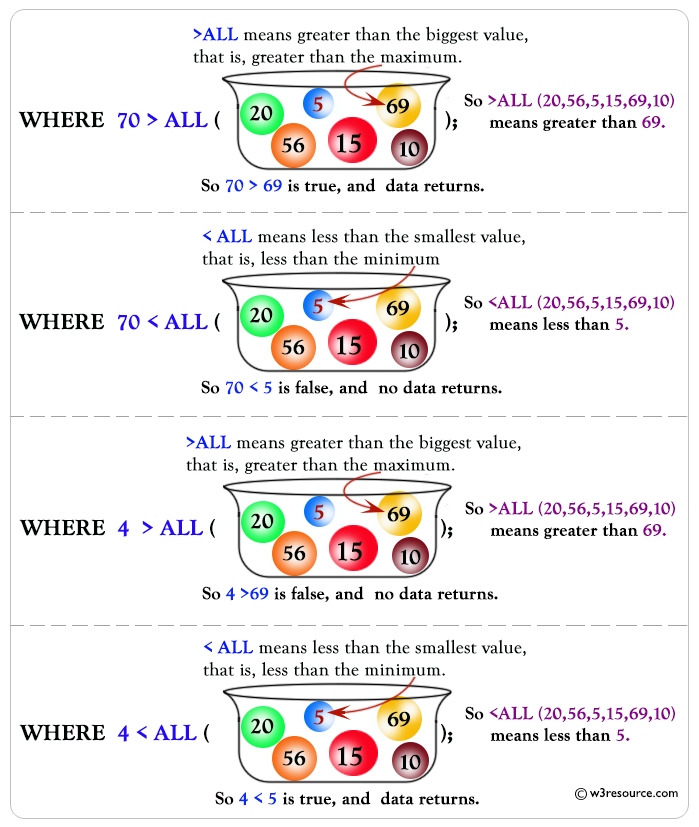# SQL ALL Operator

## ALL Operator

ALL is used to select all records of a SELECT STATEMENT. It compares a value to every value in a list or results from a query. The ALL must be preceded by the comparison operators and evaluates to TRUE if the query returns no rows. For example, ALL means greater than every value, means greater than the maximum value. Suppose ALL (1, 2, 3) means greater than 3.

Syntax:

```SELECT [column_name... | expression1 ]
FROM [table_name]
WHERE expression2 comparison_operator {ALL | ANY | SOME} ( subquery )
```

Parameters:

Name Description
column_name Name of the column of the table.
expression1 Expression made up of a single constant, variable, scalar function, or column name and can also be the pieces of a SQL query that compare values against other values or perform arithmetic calculations.
table_name Name of the table.
WHERE expression2 Compares a scalar expression, such as a column against every value in the subquery for ALL operator. All rows must match the expression to return a Boolean TRUE value for the ALL operator.
comparison_operator Compares the expression to the subquery. The comparison must be a standard comparison operator (=, <>, !=, >, >=, <, or <=.

Pictorical Presentation: SQL ALL OperatorDBMS Support: ALL Operator

 DBMS Command MySQL Supported PostgreSQL Supported SQL Server Supported Oracle Supported

Example: SQL ALL operator

To get 'des_date','des_amount' and 'ord_amount' columns from the 'despatch' table with following conditions -

1. 'des_amount' of 'despatch' table is more than 'ord_amount' from 'orders' table which satisfies the condition bellow:

a) 'ord_amount' must be equal to 2000,

the following SQL statement can be used:

``````SELECT des_date,des_amount,ord_amount
FROM despatch
WHERE des_amount>ALL(
SELECT ord_amount FROM orders
WHERE ord_amount=2000);``````

Sample table: orders

Sample table: despatch

Explanation:

The inner query returns 'ord_amount' from 'orders' table for 'ord_amount' is equal to 2000
The outer query select 'des_date', 'des_amount' and 'ord_amount' form 'despatch' table whose 'des_amount' is greater than all the 'ord_amount' from 'orders' table whose 'ord_amount' is equal to 2000.

Output:

```DES_DATE  DES_AMOUNT ORD_AMOUNT
--------- ---------- ----------
12-JAN-08       3800       4000
19-OCT-08       4000       4000
24-JUL-08       4500       3500
```

See our Model Database

Practice SQL Exercises